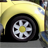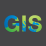# Pixel Types

298
3
01-03-2013 08:41 AMNew Contributor III
Dear all,

there is a raster layer I would like to convert to polygons witrh the tool "Raster To Polygon". But that doesn't work. If I got it right the pixel type must be "integer", but in fact it is "floating point".

With the tool "Copy Raster" I wanted to change the pixel type. There are a lot of pixel types available, but several tests resulted in raster images different from the original.

How do I know which pixel type to choose and what do I have to observe to get a raster image identical to the original?

Thanks in advance and kind regards,
Matthias
Tags (3)
1 Solution

Accepted SolutionsbyEsri Esteemed Contributor
Hi Matthias,

If you have 3D or Spatial Analyst, use the Int tool to convert the floating point raster to an integer raster.  The decimal values will be dropped from each pixel.

If you need to maintain the decimal values, first use the Times tool and multiply the raster by a constant to preserve the number of decimals.  For example, if you need to maintain 3 decimals, multiply the raster by 1000.  Then, execute the Int tool, followed by the Raster to Polygon tool.

Within your polygon shapefile/feature class you will have a Value field.  You can then use the field calculator to divide by the constant used for the Times tool, and you will then have the original pixel values.
3 RepliesbyEsri Esteemed Contributor
Hi Matthias,

If you have 3D or Spatial Analyst, use the Int tool to convert the floating point raster to an integer raster.  The decimal values will be dropped from each pixel.

If you need to maintain the decimal values, first use the Times tool and multiply the raster by a constant to preserve the number of decimals.  For example, if you need to maintain 3 decimals, multiply the raster by 1000.  Then, execute the Int tool, followed by the Raster to Polygon tool.

Within your polygon shapefile/feature class you will have a Value field.  You can then use the field calculator to divide by the constant used for the Times tool, and you will then have the original pixel values.New Contributor III
Dear Jake,by